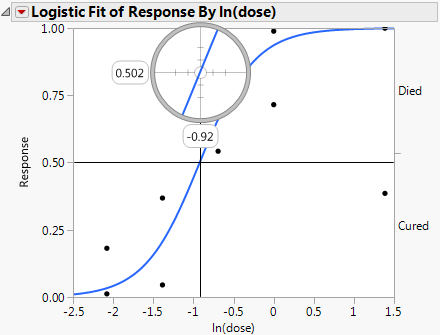Basic Analysis > Logistic Analysis > Additional Examples of Logistic Regression > Example of Inverse Prediction Using the Crosshair Tool
Publication date: 11/10/2021

## Example of Inverse Prediction Using the Crosshair Tool

This example shows you how to use the crosshair tool in JMP to visually approximate an inverse prediction. In a study of rabbits who were given penicillin, you want to know what dose of penicillin results in a 0.5 probability of curing a rabbit. In this case, the inverse prediction for 0.5 is called the ED50, the effective dose corresponding to a 50% survival rate.

Determine which dose is equally likely either to cure or to be lethal.

1. Select Help > Sample Data Library and open Penicillin.jmp.

2. Select Analyze > Fit Y by X.

3. Select Response and click Y, Response.

4. Select ln(dose) and click X, Factor.

Notice that JMP automatically fills in Count for Freq. Count was previously assigned the role of Freq.

5. From the Target Level list, select Cured.

6. Click OK.

7. Click the crosshairs tool.

8. Place the horizontal crosshair line at about 0.5 on the vertical (Response) probability axis.

9. Move the cross-hair intersection to the prediction line, and read the In(dose) value that shows on the horizontal axis.

In this example, a rabbit with a ln(dose)of approximately -0.9 is equally likely to be cured as it is to die.

Figure 8.10 Example of Crosshair Tool on Logistic Plot